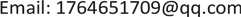﻿ 带有奇异吸收项的离散的p, ω-Laplacian方程解的淬灭现象 Quenching of the Solution of the Discrete p, ω-Laplacian Equation with Absorption Singularity Term on Graphs

Pure Mathematics
Vol.08 No.04(2018), Article ID:26021,9 pages
10.12677/PM.2018.84058

Quenching of the Solution of the Discrete p, ω-Laplacian Equation with Absorption Singularity Term on Graphs

Yafeng Li, Qiao Xin

College of Mathematics and Statistics, Yili Normal University, Yining XinjiangReceived: Jun. 28th, 2018; accepted: Jul. 16th, 2018; published: Jul. 23rd, 2018ABSTRACT

This paper mainly studies the quenching of solution of the discrete p, ω-Laplacian equation with absorption singularity term and positive Dirichlet boundary conditions. First, the local existence and uniqueness of solutions are obtained by Banach fixed point theorem. And then, on some suitable conditions, we prove that the solution quenches in finite time by comparison principle. Moreover, the upper of quenching time to solution is also obtained.

Keywords:Graph, p, ω-Laplacian Operator, Quenching, Comparison PrincipleCopyright © 2018 by authors and Hans Publishers Inc.

This work is licensed under the Creative Commons Attribution International License (CC BY).

http://creativecommons.org/licenses/by/4.0/1. 引言

$\left\{\begin{array}{l}{u}_{t}={\Delta }_{p,\omega }u-{u}^{-q},\text{\hspace{0.17em}}\text{\hspace{0.17em}}\text{\hspace{0.17em}}x\in S×\left(0,T\right),\\ u\left(x,t\right)=1,\text{\hspace{0.17em}}\text{\hspace{0.17em}}\text{\hspace{0.17em}}\text{\hspace{0.17em}}\text{\hspace{0.17em}}\text{\hspace{0.17em}}\text{\hspace{0.17em}}\text{\hspace{0.17em}}\text{\hspace{0.17em}}\text{\hspace{0.17em}}\text{\hspace{0.17em}}x\in \partial S,\\ u\left(x,0\right)={u}_{0}\left(x\right),\text{\hspace{0.17em}}\text{\hspace{0.17em}}\text{\hspace{0.17em}}x\in S,\end{array}$ (1.1)

${\Delta }_{p,\omega }u\left(x\right)=\sum _{y\in V}{|u\left(y\right)-u\left(x\right)|}^{p-2}\left[u\left(y\right)-u\left(x\right)\right]\omega \left(x,y\right),$

${\int }_{G}u=\sum _{x\in V}u\left(x\right).$

${u}_{t}\left(x,t\right)={\Delta }_{p,\omega }u\left(x,t\right)+\lambda {|u\left(x,t\right)|}^{q-1`}u\left(x,t\right),$ (1.2)

$\left\{\begin{array}{l}{u}_{t}\left(x,t\right)={\Delta }_{p,\omega }u\left(x,t\right)-{u}^{q},\text{\hspace{0.17em}}\text{\hspace{0.17em}}\left(x,t\right)\in G×\left(0,+\infty \right),\\ u\left(x,t\right)=0,\text{\hspace{0.17em}}\text{\hspace{0.17em}}\text{\hspace{0.17em}}\text{\hspace{0.17em}}\text{\hspace{0.17em}}\text{\hspace{0.17em}}\text{\hspace{0.17em}}\text{\hspace{0.17em}}\text{\hspace{0.17em}}\text{\hspace{0.17em}}\text{\hspace{0.17em}}\text{\hspace{0.17em}}\text{\hspace{0.17em}}\text{\hspace{0.17em}}\text{\hspace{0.17em}}\text{\hspace{0.17em}}\text{\hspace{0.17em}}\text{\hspace{0.17em}}\text{\hspace{0.17em}}\text{\hspace{0.17em}}\text{\hspace{0.17em}}\text{\hspace{0.17em}}\text{\hspace{0.17em}}x\in \partial G,\text{\hspace{0.17em}}t>0,\\ u\left(x,0\right)={u}_{0}\left(x\right),\text{\hspace{0.17em}}\text{\hspace{0.17em}}\text{\hspace{0.17em}}\text{\hspace{0.17em}}\text{\hspace{0.17em}}\text{\hspace{0.17em}}\text{\hspace{0.17em}}\text{\hspace{0.17em}}\text{ }\text{ }\text{\hspace{0.17em}}\text{\hspace{0.17em}}\text{\hspace{0.17em}}\text{\hspace{0.17em}}\text{\hspace{0.17em}}\text{\hspace{0.17em}}\text{\hspace{0.17em}}x\in G,\end{array}$

$\left\{\begin{array}{l}{u}_{t}\left(x,t\right)={\Delta }_{\omega }u-\lambda {u}^{-q},\text{\hspace{0.17em}}\text{\hspace{0.17em}}\text{\hspace{0.17em}}\left(x,t\right)\in S×\left(0,T\right),\\ u\left(x,t\right)=1,\text{\hspace{0.17em}}\text{\hspace{0.17em}}\text{\hspace{0.17em}}\text{\hspace{0.17em}}\text{\hspace{0.17em}}\text{\hspace{0.17em}}\text{\hspace{0.17em}}\text{\hspace{0.17em}}\text{\hspace{0.17em}}\text{\hspace{0.17em}}\text{\hspace{0.17em}}\text{\hspace{0.17em}}\text{\hspace{0.17em}}\text{\hspace{0.17em}}\text{\hspace{0.17em}}\text{\hspace{0.17em}}\text{\hspace{0.17em}}\text{ }\text{\hspace{0.17em}}\text{ }\left(x,t\right)\in \partial S×\left(0,T\right),\\ u\left(x,0\right)={u}_{0}\left(x\right),\text{\hspace{0.17em}}\text{\hspace{0.17em}}\text{\hspace{0.17em}}\text{\hspace{0.17em}}\text{\hspace{0.17em}}\text{\hspace{0.17em}}\text{\hspace{0.17em}}\text{\hspace{0.17em}}\text{\hspace{0.17em}}\text{\hspace{0.17em}}\text{\hspace{0.17em}}x\in S,\end{array}$

2. 局部解的存在与唯一性

$\left({|a|}^{p-2}a-{|b|}^{p-2}b\right)\le {C}_{1}|a-b|{\left(|a|+|b|\right)}^{p-2},$ (2.1)

$\left({|a|}^{p-2}a-{|b|}^{p-2}b\right)\left(a-b\right)\ge {C}_{2}{|a-b|}^{2}{\left(|a|+|b|\right)}^{p-2},$ (2.2)

${X}_{0}=\left\{u\in C\left[0,{t}_{0}\right]×C\left(V\right),\text{\hspace{0.17em}}\alpha \le u\le 1且对于任意\text{\hspace{0.17em}}x\in \partial S,\text{\hspace{0.17em}}u\left(x,t\right)=1\right\},$

${‖u\left(x,t\right)‖}_{{X}_{0}}=\underset{x\in V}{\mathrm{max}}\underset{t\in \left[0,{t}_{0}\right]}{\mathrm{max}}|u\left(x,t\right)|,$

${T}_{{u}_{0}}\left[u\right]\left(x,t\right)=\left\{\begin{array}{l}{u}_{0}\left(x\right)+{\int }_{0}^{t}{\Delta }_{p,\omega }u\left(x,s\right)\text{d}s-\lambda {\int }_{0}^{t}{u}^{-q}\left(x,s\right)\text{d}s,\text{\hspace{0.17em}}x\in S,\text{\hspace{0.17em}}0\le t\le {t}_{0},\\ 1,\text{\hspace{0.17em}}\text{\hspace{0.17em}}\text{\hspace{0.17em}}\text{\hspace{0.17em}}\text{\hspace{0.17em}}\text{\hspace{0.17em}}\text{\hspace{0.17em}}\text{\hspace{0.17em}}\text{\hspace{0.17em}}\text{\hspace{0.17em}}\text{\hspace{0.17em}}\text{\hspace{0.17em}}\text{\hspace{0.17em}}\text{\hspace{0.17em}}\text{\hspace{0.17em}}\text{\hspace{0.17em}}\text{\hspace{0.17em}}\text{\hspace{0.17em}}\text{\hspace{0.17em}}\text{\hspace{0.17em}}\text{\hspace{0.17em}}\text{\hspace{0.17em}}\text{\hspace{0.17em}}\text{\hspace{0.17em}}\text{\hspace{0.17em}}\text{\hspace{0.17em}}\text{\hspace{0.17em}}\text{\hspace{0.17em}}\text{\hspace{0.17em}}\text{\hspace{0.17em}}\text{\hspace{0.17em}}\text{\hspace{0.17em}}\text{\hspace{0.17em}}\text{\hspace{0.17em}}\text{\hspace{0.17em}}\text{\hspace{0.17em}}\text{\hspace{0.17em}}\text{\hspace{0.17em}}\text{\hspace{0.17em}}\text{\hspace{0.17em}}\text{\hspace{0.17em}}\text{\hspace{0.17em}}\text{\hspace{0.17em}}\text{\hspace{0.17em}}\text{\hspace{0.17em}}\text{\hspace{0.17em}}\text{\hspace{0.17em}}\text{\hspace{0.17em}}\text{\hspace{0.17em}}\text{\hspace{0.17em}}\text{\hspace{0.17em}}\text{\hspace{0.17em}}\text{\hspace{0.17em}}\text{\hspace{0.17em}}\text{\hspace{0.17em}}\text{\hspace{0.17em}}\text{\hspace{0.17em}}\text{\hspace{0.17em}}\text{ }\text{ }x\in \partial S,\text{\hspace{0.17em}}0\le t\le {t}_{0},\end{array}$

${‖{T}_{{u}_{0}}\left[u\right]-{T}_{{v}_{0}}\left[v\right]‖}_{{X}_{0}}\le {‖{u}_{0}-{v}_{0}‖}_{\infty }+Ct{‖u-v‖}_{{X}_{0}},$

$\begin{array}{l}|{T}_{{u}_{0}}\left[u\right]\left(x,{t}_{1}\right)-{T}_{{u}_{0}}\left[u\right]\left(x,{t}_{2}\right)|\\ \le |{\int }_{{t}_{1}}^{{t}_{2}}\sum _{y\in V}\omega \left(x,y\right)\left({|u\left(y,s\right)-u\left(x,s\right)|}^{p-2}\left[u\left(y,s\right)-u\left(x,s\right)\right]\right)\text{d}s|+\lambda |{\int }_{{t}_{1}}^{{t}_{2}}{u}^{-q}\left(x,s\right)\text{d}s|\\ \le \left({t}_{2}-{t}_{1}\right)\left(2{‖u‖}_{{X}_{0}}^{p-1}\underset{x\in S}{\mathrm{max}}\left({d}_{\omega }x\right)+\lambda {‖u‖}_{{X}_{0}}^{-q}\right),\end{array}$

$\begin{array}{c}{T}_{{u}_{0}}\left[u\right]\left(x,t\right)={u}_{0}\left(x\right)+{\int }_{0}^{t}\sum _{y\in V}{|u\left(y,s\right)-u\left(x,s\right)|}^{p-2}u\left(y,s\right)\omega \left(x,y\right)\text{d}s\\ \text{\hspace{0.17em}}\text{\hspace{0.17em}}-{\int }_{0}^{t}\sum _{y\in V}{|u\left(y,s\right)-u\left(x,s\right)|}^{p-2}u\left(x,s\right)\omega \left(x,y\right)\text{d}s-\lambda {\int }_{0}^{t}{u}^{-q}\left(x,s\right)\text{d}s\\ \ge {u}_{0}\left(x\right)-{\int }_{0}^{t}\sum _{y\in V}{|u\left(y,s\right)-u\left(x,s\right)|}^{p-2}u\left(x,s\right)\omega \left(x,y\right)\text{d}s-\lambda {\int }_{0}^{t}{u}^{-q}\left(x,s\right)\text{d}s\\ \ge 2\alpha -\left(|V|\mathrm{max}\left({d}_{\omega }x\right){\left(1-\alpha \right)}^{p-2}+\lambda {\alpha }^{-q}\right){t}_{0}\ge \alpha ,\end{array}$

$\begin{array}{c}{T}_{{u}_{0}}\left[u\right]\left(x,t\right)={u}_{0}\left(x\right)+{\int }_{0}^{t}\sum _{y\in V}{|u\left(y,s\right)-u\left(x,s\right)|}^{p-2}u\left(y,s\right)\omega \left(x,y\right)\text{d}s\\ \text{\hspace{0.17em}}\text{\hspace{0.17em}}-{\int }_{0}^{t}\sum _{y\in V}{|u\left(y,s\right)-u\left(x,s\right)|}^{p-2}u\left(x,s\right)\omega \left(x,y\right)\text{d}s-\lambda {\int }_{0}^{t}{u}^{-q}\left(x,s\right)\text{d}s\\ \le {u}_{0}\left(x\right)+{\int }_{0}^{t}\sum _{y\in V}{|u\left(y,s\right)-u\left(x,s\right)|}^{p-2}u\left(y,s\right)\omega \left(x,y\right)\text{d}s\\ \le {u}_{0}\left(x\right)+{t}_{0}|V|\mathrm{max}\left({d}_{\omega }x\right){\left(1-\alpha \right)}^{p-2}\le 1.\end{array}$

$\begin{array}{l}|{T}_{{u}_{0}}\left[u\right]\left(x,t\right)-{T}_{{v}_{0}}\left[v\right]\left(x,t\right)|\\ \le {‖{u}_{0}-{v}_{0}‖}_{\infty }+|{\int }_{0}^{t}\sum _{y\in V}\omega \left(x,y\right)\left({|u\left(y,s\right)-u\left(x,s\right)|}^{p-2}\left[u\left(y,s\right)-u\left(x,s\right)\right]\\ \text{\hspace{0.17em}}\text{\hspace{0.17em}}\text{ }-{|v\left(y,s\right)-v\left(x,s\right)|}^{p-2}\left[v\left(y,s\right)-v\left(x,s\right)\right]\right)\text{d}s|+\lambda |{\int }_{0}^{t}\left[{u}^{-q}-{v}^{-q}\right]\text{d}s|\end{array}$

$\begin{array}{l}\le {‖{u}_{0}-{v}_{0}‖}_{\infty }+c{\int }_{0}^{t}\sum _{y\in V}\omega \left(x,y\right){|u\left(y,s\right)-u\left(y,s\right)-v\left(y,s\right)+v\left(y,s\right)|}^{p-2}\\ \text{\hspace{0.17em}}\text{\hspace{0.17em}}\text{ }×\left(|u\left(y,s\right)-u\left(x,s\right)|+|v\left(y,s\right)-v\left(x,s\right)|\right)\text{d}s+\lambda |{\int }_{0}^{t}\left[{u}^{-q}-{v}^{-q}\right]\text{d}s|\\ \le {‖{u}_{0}-{v}_{0}‖}_{\infty }+t\left(\lambda q{\eta }^{-q-1}+2c{\left(4\eta \right)}^{p-2}|V|\right){‖u-v‖}_{{X}_{0}},\end{array}$

${‖{T}_{{u}_{0}}\left[u\right]\left(x,t\right)-{T}_{{v}_{0}}\left[v\right]\left(x,t\right)‖}_{{X}_{0}}\le {‖{u}_{0}-{v}_{0}‖}_{\infty }+Ct{‖u-v‖}_{{X}_{0}},$

$\underset{t\to T}{\mathrm{lim}}u\left(x,t\right)=0.$

$u\left(x,t\right)=\left\{\begin{array}{l}{u}_{0}\left(x\right)+{\int }_{0}^{t}{\Delta }_{p,\omega }u\left(x,s\right)\text{d}s-\lambda {\int }_{0}^{t}{u}^{-q}\left(x,s\right)\text{d}s,\text{\hspace{0.17em}}\text{\hspace{0.17em}}x\in S,\text{\hspace{0.17em}}0\le t\le {t}_{0},\\ 1,\text{\hspace{0.17em}}\text{\hspace{0.17em}}\text{\hspace{0.17em}}\text{\hspace{0.17em}}\text{\hspace{0.17em}}\text{\hspace{0.17em}}\text{\hspace{0.17em}}\text{\hspace{0.17em}}\text{\hspace{0.17em}}\text{\hspace{0.17em}}\text{\hspace{0.17em}}\text{\hspace{0.17em}}\text{\hspace{0.17em}}\text{\hspace{0.17em}}\text{\hspace{0.17em}}\text{\hspace{0.17em}}\text{\hspace{0.17em}}\text{\hspace{0.17em}}\text{\hspace{0.17em}}\text{\hspace{0.17em}}\text{\hspace{0.17em}}\text{\hspace{0.17em}}\text{\hspace{0.17em}}\text{\hspace{0.17em}}\text{\hspace{0.17em}}\text{\hspace{0.17em}}\text{\hspace{0.17em}}\text{\hspace{0.17em}}\text{\hspace{0.17em}}\text{\hspace{0.17em}}\text{\hspace{0.17em}}\text{ }\text{\hspace{0.17em}}\text{\hspace{0.17em}}\text{\hspace{0.17em}}\text{\hspace{0.17em}}\text{\hspace{0.17em}}\text{\hspace{0.17em}}\text{\hspace{0.17em}}\text{\hspace{0.17em}}\text{\hspace{0.17em}}\text{\hspace{0.17em}}\text{\hspace{0.17em}}\text{\hspace{0.17em}}\text{\hspace{0.17em}}\text{\hspace{0.17em}}\text{\hspace{0.17em}}\text{\hspace{0.17em}}\text{\hspace{0.17em}}\text{\hspace{0.17em}}\text{\hspace{0.17em}}\text{\hspace{0.17em}}\text{\hspace{0.17em}}\text{\hspace{0.17em}}\text{\hspace{0.17em}}\text{\hspace{0.17em}}\text{\hspace{0.17em}}\text{\hspace{0.17em}}\text{\hspace{0.17em}}\text{\hspace{0.17em}}x\in \partial S,\text{\hspace{0.17em}}0\le t\le {t}_{0},\end{array}$

3. 比较原理

$\left\{\begin{array}{l}{\overline{u}}_{t}\ge {\Delta }_{p,\omega }\overline{u}-\lambda {\overline{u}}^{-q},\text{\hspace{0.17em}}\text{ }x\in S,\text{\hspace{0.17em}}t\in \left(0,T\right),\\ \overline{u}\left(x,t\right)\ge 1,\text{\hspace{0.17em}}\text{\hspace{0.17em}}\text{\hspace{0.17em}}\text{\hspace{0.17em}}\text{\hspace{0.17em}}\text{\hspace{0.17em}}\text{\hspace{0.17em}}\text{\hspace{0.17em}}\text{\hspace{0.17em}}\text{\hspace{0.17em}}\text{\hspace{0.17em}}\text{\hspace{0.17em}}x\in \partial S,\text{\hspace{0.17em}}t\in \left(0,T\right),\\ \overline{u}\left(x,0\right)\ge {u}_{0}\left(x\right),\text{\hspace{0.17em}}\text{\hspace{0.17em}}\text{\hspace{0.17em}}\text{\hspace{0.17em}}x\in S,\end{array}$

$U\left(x,t\right)=\underset{_}{u}\left(x,t\right)-\overline{u}\left(x,t\right)$ ，显然 $x\in S,U\left(x,0\right)<0$ ，那么通过上解与下解的定义可得

${U}_{t}\le \left({\Delta }_{p,\omega }\underset{_}{u}\left(x,t\right)-{\Delta }_{p,\omega }\overline{u}\left(x,t\right)\right)-\lambda \left({\underset{_}{u}}^{-q}\left(x,t\right)-{\overline{u}}^{-q}\left(x,t\right)\right),\text{\hspace{0.17em}}\text{\hspace{0.17em}}\left(x,t\right)\in S×\left(0,T\right).$ (3.1)

${U}_{+}\left(x,t\right)=\mathrm{max}\left\{U\left(x,t\right),0\right\}$ ，现在不等式(3.1)两边同乘 ${U}_{+}$ 并在S积分

$\frac{1}{2}{\int }_{x\in S}{\left({U}_{+}^{2}\left(x,t\right)\right)}_{t}\le {\int }_{x\in S}{U}_{+}\left(x,t\right)\left[\left({\Delta }_{p,\omega }\underset{_}{u}\left(x,t\right)-{\Delta }_{p,\omega }\overline{u}\left(x,t\right)\right)-\lambda \left({\underset{_}{u}}^{-q}\left(x,t\right)-{\overline{u}}^{-q}\left(x,t\right)\right)\right],$ (3.2)

$\begin{array}{l}{F}_{p,\omega }\left(\overline{u},\underset{_}{u}\right)\left(x,y,t\right)=\omega \left(x,y\right)\left\{{|\underset{_}{u}\left(y,t\right)-\underset{_}{u}\left(x,t\right)|}^{p-2}\left(\underset{_}{u}\left(y,t\right)-\underset{_}{u}\left(x,t\right)\right)\\ \text{\hspace{0.17em}}\text{\hspace{0.17em}}\text{\hspace{0.17em}}\text{\hspace{0.17em}}\text{\hspace{0.17em}}\text{\hspace{0.17em}}\text{\hspace{0.17em}}\text{\hspace{0.17em}}\text{\hspace{0.17em}}\text{\hspace{0.17em}}\text{\hspace{0.17em}}\text{\hspace{0.17em}}\text{\hspace{0.17em}}\text{\hspace{0.17em}}\text{\hspace{0.17em}}\text{\hspace{0.17em}}\text{\hspace{0.17em}}\text{\hspace{0.17em}}\text{\hspace{0.17em}}\text{\hspace{0.17em}}\text{\hspace{0.17em}}\text{\hspace{0.17em}}\text{\hspace{0.17em}}\text{\hspace{0.17em}}\text{\hspace{0.17em}}\text{\hspace{0.17em}}\text{\hspace{0.17em}}\text{\hspace{0.17em}}-{|\overline{u}\left(y,t\right)-\overline{u}\left(x,t\right)|}^{p-2}\left(\overline{u}\left(y,t\right)-\overline{u}\left(x,t\right)\right)\right\},\end{array}$

${F}_{p,\omega }\left(\overline{u},\underset{_}{u}\right)\left(x,y,t\right)\left[U\left(y,t\right)-U\left(x,t\right)\right]\ge 0,$ (3.3)

$\begin{array}{l}{\int }_{S}U\left(x,t\right)\left({\Delta }_{p,\omega }\underset{_}{u}\left(x,t\right)-{\Delta }_{p,\omega }\overline{u}\left(x,t\right)\right)\\ =\sum _{x\in J\left(t\right)}\sum _{y\in V}U\left(x,t\right){F}_{p,\omega }\left(\overline{u},\underset{_}{u}\right)\left(x,y,t\right)\\ =\sum _{x\in J\left(t\right)}\sum _{y\in J\left(t\right)}U\left(x,t\right){F}_{p,\omega }\left(\overline{u},\underset{_}{u}\right)\left(x,y,t\right)\\ \text{\hspace{0.17em}}\text{\hspace{0.17em}}\text{ }+\sum _{x\in J\left(t\right)}\sum _{y\in V\J\left(t\right)}U\left(x,t\right){F}_{p,\omega }\left(\overline{u},\underset{_}{u}\right)\left(x,y,t\right)\text{\hspace{0.17em}}.\end{array}$

$\begin{array}{l}\sum _{x\in J\left(t\right)}\sum _{y\in J\left(t\right)}U\left(x,t\right){F}_{p,\omega }\left(\overline{u},\underset{_}{u}\right)\left(x,y,t\right)\\ =\frac{1}{2}\sum _{x\in J\left(t\right)}\sum _{y\in J\left(t\right)}U\left(x,t\right){F}_{p,\omega }\left(\overline{u},\underset{_}{u}\right)\left(x,y,t\right)+\frac{1}{2}\sum _{x\in J\left(t\right)}\sum _{y\in J\left(t\right)}U\left(x,t\right){F}_{p,\omega }\left(\overline{u},\underset{_}{u}\right)\left(x,y,t\right)\\ =\frac{1}{2}\sum _{x\in J\left(t\right)}\sum _{y\in J\left(t\right)}U\left(x,t\right){F}_{p,\omega }\left(\overline{u},\underset{_}{u}\right)\left(x,y,t\right)-\frac{1}{2}\sum _{y\in J\left(t\right)}\sum _{x\in J\left(t\right)}U\left(y,t\right){F}_{p,\omega }\left(\overline{u},\underset{_}{u}\right)\left(x,y,t\right)\\ =-\frac{1}{2}\sum _{x\in J\left(t\right)}\sum _{y\in J\left(t\right)}\left(U\left(y,t\right)-U\left(x,t\right)\right){F}_{p,\omega }\left(\overline{u},\underset{_}{u}\right)\left(x,y,t\right)\le 0,\end{array}$ (3.4)

$\begin{array}{l}\sum _{x\in J\left(t\right)}\sum _{y\in V\J\left(t\right)}U\left(x,t\right){F}_{p,\omega }\left(\overline{u},\underset{_}{u}\right)\left(x,y,t\right)\\ =\sum _{x\in J\left(t\right)}\sum _{y\in V\J\left(t\right)}U\left(x,t\right)\frac{1}{U\left(y,t\right)-U\left(x,t\right)}\left(U\left(y,t\right)-U\left(x,t\right)\right){F}_{p,\omega }\left(\overline{u},\underset{_}{u}\right)\left(x,y,t\right)<0,\end{array}$ (3.5)

${\underset{_}{u}}^{-q}\left(x,t\right)-{\overline{u}}^{-q}\left(x,t\right)=-q{\xi }^{-q-1}\left(x,t\right)U\left(x,t\right),$

${\int }_{x\in S}U\left(x,t\right)\left({\underset{_}{u}}^{-q}\left(x,t\right)-{\overline{u}}^{-q}\left(x,t\right)\right)\le -{m}^{-q-1}\lambda {\int }_{x\in S}{\left({U}_{+}\left(x,t\right)\right)}^{2},$ (3.6)

${\int }_{x\in S}{\left({\left({U}_{+}\left(x,t\right)\right)}^{2}\right)}_{t}<\lambda {m}^{-q-1}{\int }_{x\in S}{\left({U}_{+}\left(x,t\right)\right)}^{2},$

$L\left(t\right)={\int }_{x\in S}{\left({U}_{+}\left(x,t\right)\right)}^{2}$ ，我们有 ${L}^{\prime }\left(t\right) 这里 $k=\lambda {m}^{-q-1}$ ，因为 ${U}_{+}\left(x,0\right)=0$ ，而且 $L\left(0\right)=0$ ，因此，我们有 $L\left(u\right)\equiv 0$ ，于是得到 ${U}_{+}\left(x,t\right)=0$ ，因此可推出 $\overline{u}\left(x,t\right)\ge \underset{_}{u}\left(x,t\right)$

4. 淬灭现象

$\underset{x\in S}{\mathrm{max}}\underset{t\in \left(0,T\right)}{\mathrm{max}}|u\left(x,t\right)|\le \underset{x\in S}{\mathrm{max}}|{u}_{0}|,\text{\hspace{0.17em}}\text{\hspace{0.17em}}\sum _{x\in S}u\left(x,t\right)\le \sum _{x\in S}{u}_{0}\left(x\right),$

$\sum _{x\in S}{u}_{t}\left(x,t\right)=\sum _{x\in S}{\Delta }_{p,\omega }u\left(x,t\right)-\lambda \sum _{x\in S}{u}^{-q}\left(x,t\right),$ (4.1)

$F\left(x,y,t\right)=\omega \left(x,y\right){|u\left(y,t\right)-u\left(x,t\right)|}^{p-2}\left[u\left(y,t\right)-u\left(x,t\right)\right],$

$\begin{array}{c}\sum _{x\in S}{\Delta }_{p,\omega }u\left(x,t\right)=\sum _{x\in S}\sum _{y\in S}F\left(x,y,t\right)+\sum _{x\in S}\sum _{y\in \partial S}F\left(x,y,t\right)\\ =\frac{1}{2}\sum _{x\in S}\sum _{y\in S}F\left(x,y,t\right)+\frac{1}{2}\sum _{x\in S}\sum _{y\in S}F\left(x,y,t\right)+\sum _{x\in S}\sum _{y\in \partial S}F\left(x,y,t\right)\\ =\frac{1}{2}\sum _{x\in S}\sum _{y\in S}F\left(x,y,t\right)-\frac{1}{2}\sum _{y\in S}\sum _{x\in S}F\left(x,y,t\right)+\sum _{x\in S}\sum _{y\in \partial S}F\left(x,y,t\right)\\ =\sum _{x\in S}\sum _{y\in \partial S}{|u\left(y,t\right)-u\left(x,t\right)|}^{p-2}\left[u\left(y,t\right)-u\left(x,t\right)\right]\omega \left(x,y\right)\\ =\sum _{x\in S}K\left(x\right){|1-u\left(x,t\right)|}^{p-2}\left[1-u\left(x,t\right)\right]\end{array}$

$\sum _{x\in S}{u}_{t}\left(x,t\right)=\sum _{x\in S}K\left(x\right){|1-u\left(x,t\right)|}^{p-2}\left[1-u\left(x,t\right)\right]-\lambda \sum _{x\in S}{u}^{-q}\left(x,t\right)$ . (4.2)

$\sum _{x\in S}{u}_{t}\left(x,t\right)=\sum _{x\in S}K\left(x\right){\left(1-u\left(x,t\right)\right)}^{p-1}-\lambda \sum _{x\in S}{u}^{-q}\left(x,t\right),$ (4.3)

$\begin{array}{l}\frac{\text{d}}{\text{d}t}\sum _{x\in S}\frac{{\left[{\left(u\left(x,t\right)-M\right)}_{+}\right]}^{2}}{2}\\ =\sum _{x\in S}K\left(x\right){\left(1-u\left(x,t\right)\right)}^{p-1}{\left(u\left(x,t\right)-M\right)}_{+}-\lambda \sum _{x\in S}\left[{u}^{-q}\left(x,t\right)\right]{\left(u\left(x,t\right)-M\right)}_{+}\\ \le \sum _{x\in S}K{\left(u\left(x,t\right)-M\right)}_{+}-\lambda \sum _{x\in S}{u}^{-q}\left(x,t\right){\left(u\left(x,t\right)-M\right)}_{+}\\ =\sum _{x\in S}\left[K-\lambda {u}^{-q}\left(x,t\right)\right]{\left(u\left(x,t\right)-M\right)}_{+}.\end{array}$

$\frac{\text{d}}{\text{d}t}\sum _{x\in S}\frac{{\left[{\left(u\left(x,t\right)-M\right)}_{+}\right]}^{2}}{2}\le 0$ .

$A\left(t\right)=\sum _{x\in S}\frac{{\left[{\left(u\left(x,t\right)-M\right)}_{+}\right]}^{2}}{2}$ ，可得 ${A}^{\prime }\left(t\right)\le 0$$A\left(0\right)=0$ ，可得

$\sum _{x\in S}\frac{{\left[{\left(u\left(x,t\right)-M\right)}_{+}\right]}^{2}}{2}=0,$

$\underset{x\in S}{\mathrm{max}}\underset{t\in \left[0,T\right)}{\mathrm{max}}|u\left(x,t\right)|\le \underset{x\in S}{\mathrm{max}}|{u}_{0}|,$ (4.4)

$\frac{\text{d}}{\text{d}t}\sum _{x\in S}u\left(x,t\right)\le K-\lambda \sum _{x\in S}{u}^{-q}\left(x,t\right),$ (4.5)

$\sum _{x\in S}u\left(x,t\right)\le \sum _{x\in S}{u}_{0}\left(x\right),$ (4.6)

$\frac{\text{d}}{\text{d}t}\sum _{x\in S}u\left(x,t\right)\le K-\lambda |S|{M}^{-q}$ (4.7)

${\int }_{\text{0}}^{t}\eta \left(s\right)\text{d}s\le {\int }_{0}^{t}\left[K-\lambda |S|{M}^{-q}\right]\text{d}s$

$\eta \left(t\right)-\eta \left(0\right)\le \left[K-|S|{M}^{-q}\right]t,$

$T\le \frac{\eta \left(t\right)-\eta \left(0\right)}{K-|S|{M}^{-q}}\le \frac{-\eta \left(0\right)}{K-\lambda |S|{M}^{-q}}=\frac{-\sum _{x\in S}{u}_{0}\left(x\right)}{K-\lambda |S|{M}^{-q}},$

Quenching of the Solution of the Discrete p, ω-Laplacian Equation with Absorption Singularity Term on Graphs[J]. 理论数学, 2018, 08(04): 436-444. https://doi.org/10.12677/PM.2018.84058

1. 1. Chung, S.Y. and Berenstein, C.A. (2005) ω-Harmonic Functions and Inverse Conductivity Problems on Networks. SIAM Journal on Applied Mathematics, 65, 1200-1226. https://doi.org/10.1137/S0036139903432743

2. 2. Chung, S.Y. (2014) Critical Blow-Up and Global Existence for Discrete Nonlinear p-Laplacian Parabolic Equations. Discrete Dynamics in Nature and Society, 2014, Article ID: 716327. https://doi.org/10.1155/2014/716327

3. 3. Lee, Y.S. and Chung, S.Y. (2011) Extinction and Positivity of Solutions of the p-Laplacian Evolution Equation on Networks. Journal of Mathematical Analysis and Applications, 380, 642-652. https://doi.org/10.1016/j.jmaa.2011.03.006

4. 4. Xin, Q, Mu, C. and Liu, D. (2011) Extinction and Positivity of the Solution for a p-Laplacian Equation with Absorption on Graphs. Journal of Applied Mathematics, 2011, Article ID: 937079. https://doi.org/10.1155/2011/937079

5. 5. 王坤, 辛巧. 图上的p-Laplacian方程解的性质及其数值仿真[J]. 长春师范大学学报, 2016, 35(2): 9-13.

6. 6. Xin, Q. and Liu, D. (2017) Quenching for the Discrete Heat Equation with a Singular Absorption Term on Finite Graphs. Journal of Computational Analysis and Applications, 23, 1265-1280.

7. 7. Barrett, J.W. and Liu, W.B. (1994) Finite Element Approximation of the Parabolic p-Laplacian. SIAM Journal on Numerical Analysis, 31, 413-428. https://doi.org/10.1137/0731022